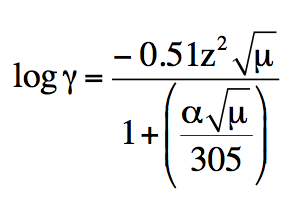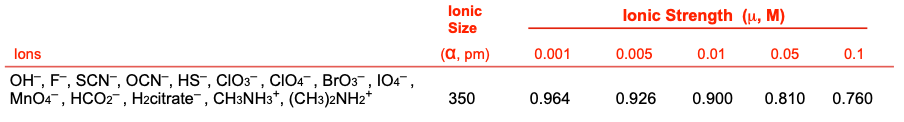Clutch Prep is now a part of Pearson
Ch.7 - Activity and the Systematic Treatment of EquilibriumWorksheetSee all chapters

# Activity Coefficients

See all sections
Sections
Ionic Strength of Soluble Salts
Activity Coefficients
pH Revisited

Activity coefficients is factor used in describing the departure from ideal behavior for a reaction mixture.

###### Activity Coefficients

Concept #1: In order to express the effect of ionic strength on the concentration of species we calculate its activity with the use of an activity coefficient, which is given in units of gamma.

Example #1: For the following compound, state the solubility product expression with its activity coefficient.

Cu2(SO4)3

Example #2: For the following compound, state the solubility product expression with its activity coefficient.

Mo2S5

###### Activity Coefficient Table

Example #3: Find the activity coefficient for the ion specified in the following compound:

Na+ in 0.005 M NaCl

Example #4: Find the activity coefficient for the ion specified in the following compound:

CN  in 1.0 mM RbCN

Example #5: Find the activity coefficient for the ion specified in the following compound:

Zr4+ in 5.0 mM Zr(NO3)4

Practice: Calculate the activity coefficient of H+ using the extended Debye-Huckel equation for a solution comprised of H+ and I . Given that H+ has a size of 9.00 x 10-10 m and the molar concentration of the solution is 0.075.

log γ = − 0.51 z 2 µ / 1 + (α µ / 305)###### Interpolation: Non-ideal Ionic Strength

Concept #2: Sometimes the ionic strength of a dissolvable compound you calculate may not be found on your chart and other means will be require to find the activity coefficient.

Example #6: Find the activity coefficient from the given ionic strength, µ, for the following ion.

Ba2+ when µ = 0.075

Practice: Find the activity coefficient from the given ionic strength, µ, for the following ion.

F   when µ = 0.0080